# WBBSE Class 7 Science Solutions Chapter 3 Atom, Molecule and Chemical Reaction

Detailed explanations in West Bengal Board Class 7 Science Book Solutions Chapter 3 Atom, Molecule and Chemical Reaction offer valuable context and analysis.

## WBBSE Class 7 Science Chapter 3 Question Answer – Atom, Molecule and Chemical Reaction

(Page no. 85)

Try to write the symbols from names of elements given below in the first two tables.

table 1

 Name of Element Symbol Aluminiun Al Nickel Ni Arsenic As Silicon Si Zinc Zn Boron B

table 2

 Name of Element Latin name Symbol Tin Stannum Sn Mercury Hydrogirum Hg Lead Plumbum PbNow we will learn the names and symbols of elements that originate from the names of famous scientists, the place of discovery, the native country of the discoverer or some planet.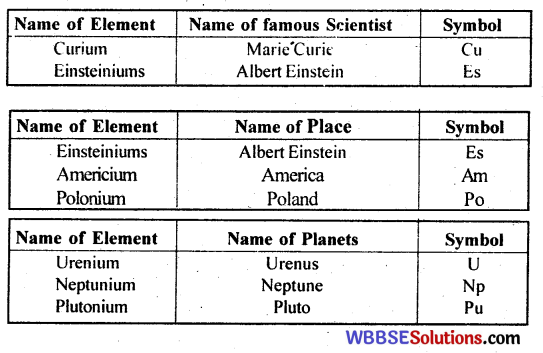(Page no. 87)

Now fill up the following table using the diagram for atomic structure of Helium atom as shown on the previous page.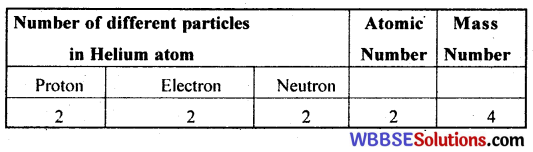Impact the diagrams above and fill up the following table :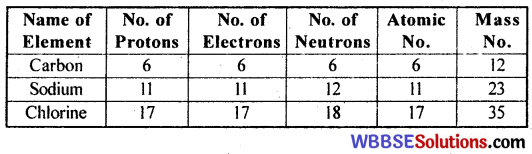(Page no. 88)

Fill up the following table with help from your teacher, if necessary.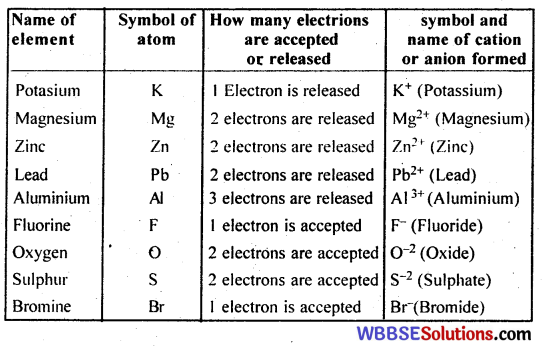(Page no. 89)

Discuss among yourselves and fill up the following table :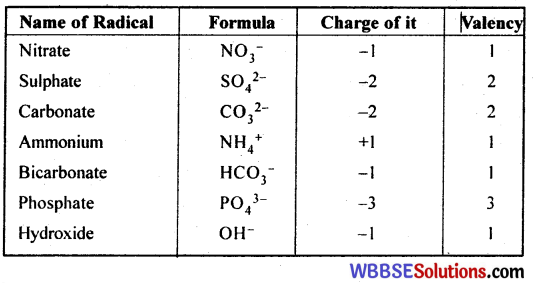Write the valencies of radicals from the number of Sodium or Potassium atom.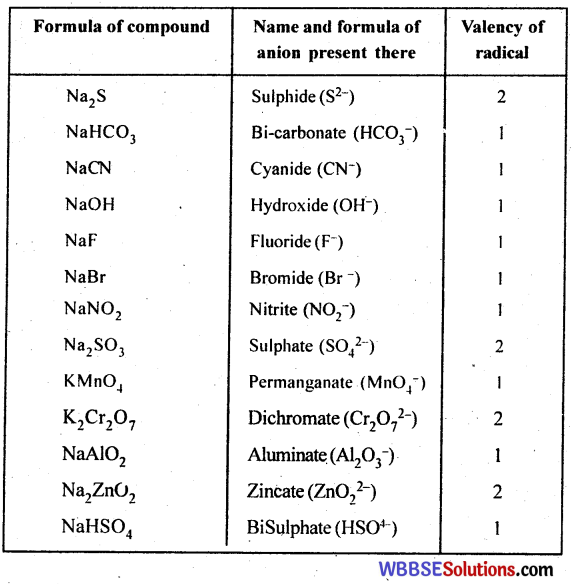(Page no. 90)

Now we shall determine the valencies of different metals and non-metals from their compounds with Chlorine. The number of Chlorine atoms which combine with an atom of an elements is the valency of that element.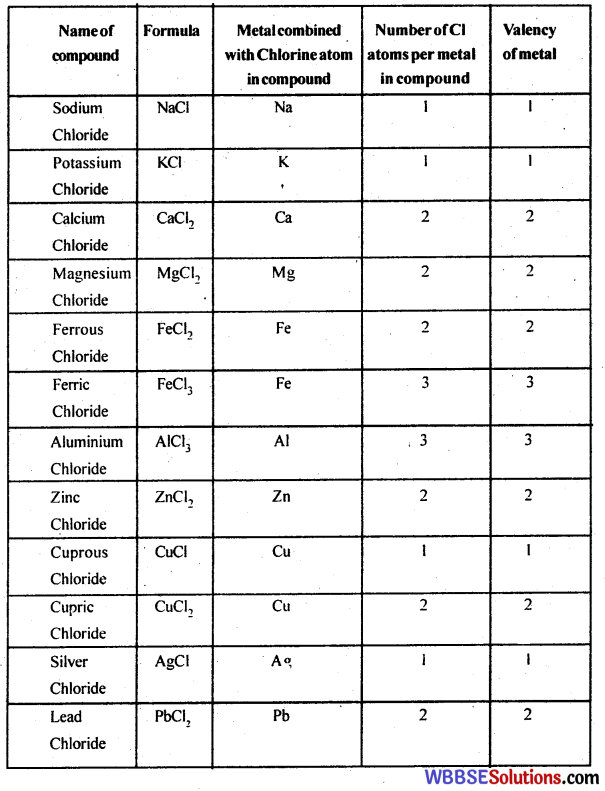(Page no. 92)

Symbols and valencies of some elements are given below. Write down the formula of the compounds by using the above procedure.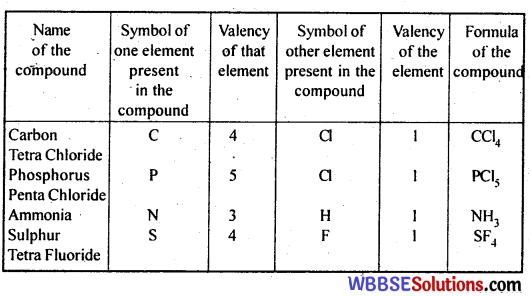Discuss among yourselves and fill up the following table using the valencies of elements and radicals.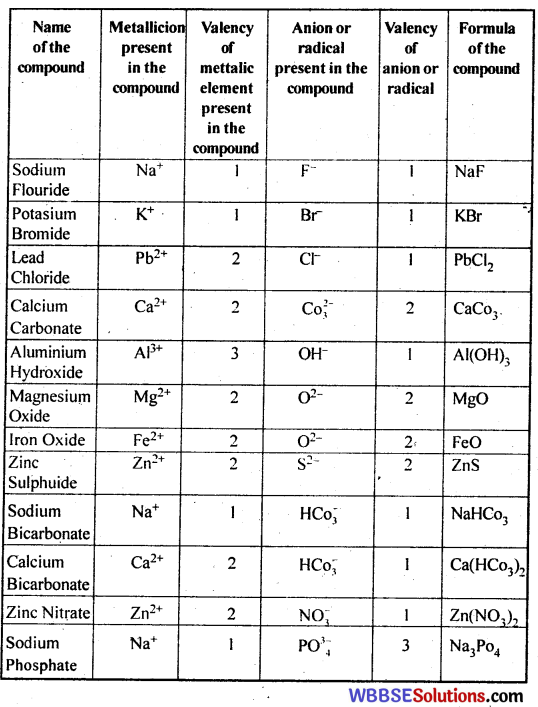(Page no. 93)

Now discuss among yourselves and fill up the following table.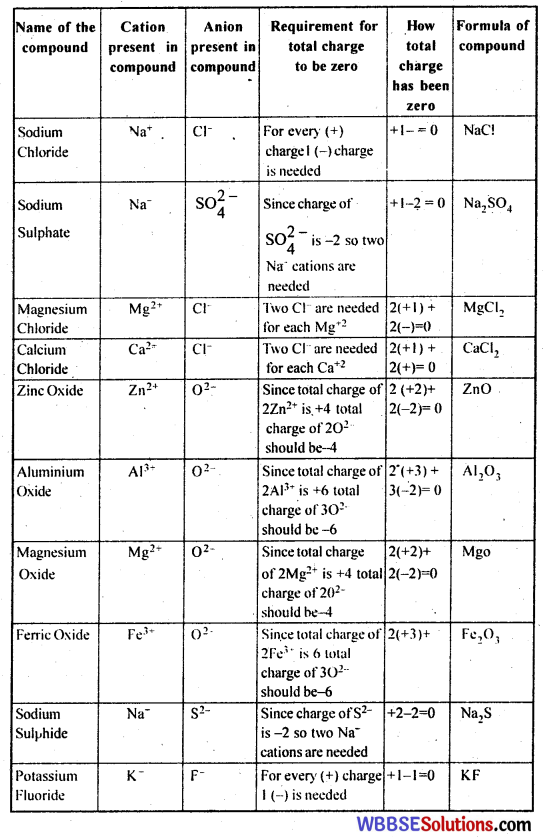(Page no. 97)

Discuss among yourselves and balance the chemical equations by putting suitable numbers in the blank places (. . .) in them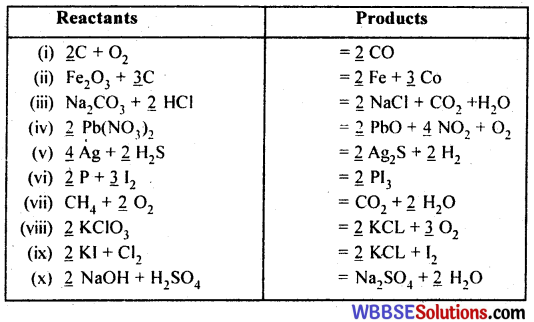Write the names and formula of products and balanced equations of the reactions by discussing among yourselves.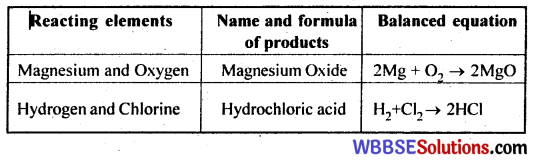Discuss anong yourselves and write the formula (e) of missing product (s) in each case.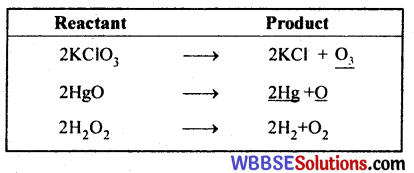(Page no. 100)

Discuss among yourselves and write the type of reaction against each equation in the table.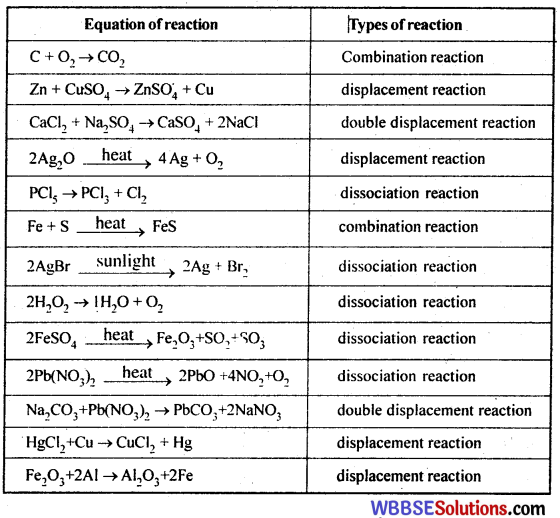### Class 7 Science Chapter 3 Question Answer West Bengal Board – Atom, Molecule and Chemical Reaction

Multiple Choice Questions & Answers : (1 mark for each question)

Question 1.
Symbol of Arsenic is-
i) Ar
ii) As
iii) An
iv) Ac
ii) As

Question 2.
Symbol of Mercury is-
i) Mc
ii) Me
iii) Mu
iv) Hg
iv) HgQuestion 3.
i) Pb
ii) Ld
iii) Le
iv) Pm
i) Pb

Question 4.
i) pbCl
ii) pbCl3
iii) pbCl2
iv) pbCl3
iii) pbCl2

Question 5.
Formula of Ferric Chloride is-
i) FeCl2
ii) FeCl3
iii) FeCl3
iv) FeCl2
ii) FeCl3

Question 6.
Formula of Potasiam Chloride-
i) KCl2
ii) KCl2
iii) PCl
iv) KCl
iv) KClQuestion 7.
Formula of Cupric Chloride-
i) CuCl2
ii) CuCl
iii) CuCl2
iv) CuCl3
i) CuCl2

Question 8.
Formula of Sodium Sulfate is-
i) NaSO
ii) NaSO4
iii) Na2 SO4
iv) Na2 SO2
iii) Na2 SO4

Question 9.
Formula of Aluminium Oxide is-
i) AlO3
ii) Al2 O3
iii) Al2 O2
iv) AlO
ii) Al2 O3Question 10.
Formula of Silver Chloride is-
i) AgCl3
ii) AgCl2
iii) Ag2 Cl
iv) AgCl
iv) AgCl

Fill in the blanks : (1 mark for each question)

1. Formula of Magnesium Chloride is ______.
MgCl2

2. The atomic number of an element is 6 and mass number is 12 then the number of neutrons in nucleus would be ______.
6.

3. In L orbit maximum _____ electrons can revolve.
8 .

4. In compound P2 O5 the valency is ______.
5.

5. Symbol of Aluminium Oxide is ______.
Al2 O3.

6. Atomic weight of carbondioxide is ______.
44.7. In chemical equation the atomic number of both sides are ______.
equal.

8. Symbol of Silver is ______.
Ag.

9. Valency of Carbon is ______.
4.

10. Mass of one electron particle is ________ of one Hydrogen atom.
1 / 1837.

State whether True or False : (1 mark for each question)

1. Hydrogen reacts with nitrogen to produce ammonia.
true2. Chemical reaction is of one type.
false

3. Ammonia is formed by the reaction of nitrogen and hydrogen.
true

4. Mercury and oxygen are produced when mercuric oxide is heated.
true

5. Formula of Ferric Oxide is Fe2 O.
false

6. Slacked lime is produced by the reaction of water with quicklime.
true7. Water is produced by chemical combination of oxygen and hydrogen.
true

8. The formula of hydrogen sulfide is H2 S2.
false

Short Questions & Answers : (1, 2 marks for each question)

Question 1.
What is the meaning of Greek word ‘Atom’?
Meaning of the word ‘Atom’ is indivisible.

Question 2.
What is atom?
The smallest particle of an element that retains the properties of the element is called an atom.

Question 3.
What is the mass number of an atom?
The total number of protons and neutrons in the nucleus of atom is called the mass number of that atom.

Question 4.
What is Atomic Number?
The number of Protons present in the nucleus of an aton of an element is known as the Atomic Number of the element.Question 5.
What is Mass Number?
The total number of Protons and Neutrons present in the nucleus of an atom of an element is known as the Mass Number of the element.

Question 6.
What is the difference between H2 and 2H ?
H2 means 2 atoms of Hydrogen and 2H mears 2 molecules of Hydrogen.

Question 7.
Write the name of an element whose atom has no neutron.
Ordinary Hydrogen atom or Protium.

Question 8.
What is the largest number of electron in K orbit?
The largest number of electron in K orbit is 2 .

Question 9.
What is the atomic number of Helium?
The atomic number of Helium is 2 .

Question 10.
How do the atoms exists in molecules?
Ator exists in combined state.Question 11.
Write the symbols of Potassium and Mercury.
Symbol of Potassium is K and Mercury is Hg.Question 12.
Who founded the theory of Molecules?

Question 13.
What is total number of Natural Element?
92.

Question 14.
What is ti.e molecular atomicity of Phosphorus.
4.Question 15.
What is tlit valency of Phosphate (PO4) ?
Valen y is 3.

Question 16.
If the orbit gues away from nucleus what would happen, will the number of electron increase 0 – decrease?
Nurber c elect:on would increase.

Question 17.
W at is :ine charge of Nucleus?
Pusitive.

Question 18.
A + B = Carbond 1 oxice, write the reacting element A and B of this reaction.
A is Carbon (C) and : 3 is Oxygen (O2)

Question 19.
Why the symbol of iron is Fe?
The Latin nam: of iron is Ferrum. So the symbol is taken as Fe.

Question 20.
What is the total number of atoms in molccule of Ammonia?
In Amonia (NH3) molecule, the number of atoms are 4.Question 21.
Which elements have zero valency?
Non-reacting elements like inert gases (He, Ni, Ar etc.) have zero valency.

Question 22.
Name the element and the radical whcih have same valency.
The element Sodium (Na) and radical Nitrate (NO3) have same valency.

Question 23.
Can we say Neutron that is the main constituent of fromation of atoms of all substances?
No, we cannot say that neutron is the main constituent.

Question 24.
What is the valency of Nitrogen in compound Ammonia?
Valency of Nitrogen is 3 in Ammonia- (NH3).

Question 25.
What is the charge of Electron?
Negative.Question 26.
When we can say an equation is balanced?
If the number of atoms of each element on the left hand side is equal to the number of atoms of that element on the right hand side, then we can say that the equation is balanced.

Long Questions & Answers : (3 marks for each question)

Question 1.
What is symbol? Give example.
When the name of an element is represented by a single letter or two letters of english alphabet it is called symbol of that element. By using symbol we can express briefly an atom. As example – symbol of Aluminium is Al, symbol of Mercury is Hg.

Question 2.
What is sign?
The way of expressing briefly the molecules of elements and compounds is called sign. For example –
Carbon-di-oxide (CO2), Sulphur-di-oxide (SO2). Nitric Oxide (NO).

Question 3.
Explain the process of writing symbols.
(1) For some elements the first letter of the name of the element written in capital. is used as Symbol.
Example: H – Hydrogen, O – Oxygen,

(2) When there are two or more than two elements whose names start with the same alphabet. the elements are given a two-letter symbol. The first letter of the symbol is the first letter of the name of the element, written in small letters.
Examples –
B – Boron, Be- Beryllium, Br- Bromine(3) The symbol of each element is written in capital letter.
Example –
Carbon -C, Oxygen -O, Nitrogen -N.
(4) The symbols of a few elements are derived from their Latin names.
Examples –
Sodium – Na (derived from latin name Natrium); Iron – Fe (latin name Ferrum).

Question 4.
What is an element?
A element is a pure substance that is made up of identical minute particles called atoms. Elemelits cannot be split into two or more simplers substances by chemical methods. There are only about 114 elements known till date, out of which 92 are found in nature.

Question 5.
What is molecule?
Atoms sometimes exist alone but more often they combine with other atoms of the same or different kind. A group of atoms formed by the combination of atoms is called a molecule. A molecule can exist by itself. A molecule may contain similar or dissimilar atoms.

Question 6.
How many particles are there in an atom?
Three types of particles are present in an atom –
i) Proton,
ii) Electron,
ii) Neutron.
(-) Indicates particles called Proton. These are charged with positive electricity or positively charged particles.
(O) Indicates particles called electrons. These are negatively charged particles.
( ) Indicates particles called Neutron. They have no electricity.Question 7.
What is nucleus? Where do the electrons revolve?
The Protons and Neutrons are grouped at a tiny place in the center of an atom. This place is called nucleus of an atom.
Electrons revolve in differenrt paths around the nucleus. The paths around the nucleus in which electrons can revolve are called orbits.

Question 8.
What are ions?
The atoms whcih attain positive or negative charges due to gain or loss of one or more electrons are called ions.
The positively charged ions formed by the loss of electrons from an atom are called cations. The negatively charged ions formed by the gain of electrons by an atom are called anions. As example Na^{+is a cation and Cl^{-is an anion.

Question 9.
Sometimes one or more than one atom of same or different elements assemble of to form ions. These are called radicals. These radicals participate in chemical reaction in such assembled condition.

Question 10.
What is Valency?
The capacity of elements to combine with each other is called their valency.
Valency is equal to the number of electrons of an atom can donate or accept to attain the stability of a complete valence shell. The elements with valency 1,2 and 3 are respectively called monovalent, divalent and trivalent element.
Example –
Valency of Sodium(Na) is 1.
Oxygen (O) is 2.
Nitrogen (N) is 3.

Question 11.
What is variable valency?
Various elements like Iron, Copper etc. possesses more than one valency. These elements can form different compounds by changing their valencies when they combine with different number of atoms of the same element. These elements have variable valencies. For example, iron forms two compounds with chlorine, FeCl2 (Ferrous Chloride) and FeCl3 ( Ferric Chloride). The valency of Iron in the two compounds is 2 and 3 respectively.Question 12.
What is a chemical equation? Define reactants and products.
If we express the chemical reaction in brief by using the formulae of reactants and products, then it is called a chemical equation.
The elements or compounds that take part in the chemical reaction are called the reactants.
The elements or compounds that form as a result of the chemical reaction are called the products.

Question 13.
How many types of chemical reactions are there?
There are four types of chemical reactions –

1. Combination reaction.
2. Dissociation reaction.
3. Displacement reaction.
4. Double displacement reaction.

Question 14.
What is combination reaction? Give an example.
Ans:
The chemical reaction in which two or more elements or compounds combine to form a single new compound is called a combination reaction.
For example :-
Water is produced by combination of oxygen and Hydrogen.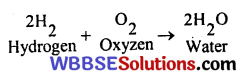Question 15.
What is dissociation reaction? Give example.
When a single compound is dissociated into more tharrone subtance by the influence of heat or electricity then such type of reaction is called dissociation reaction.
For example –
When Potassium Chlorate is heated, oxygen is liberated.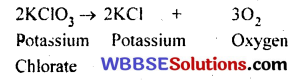Question 16.
What is Displacement reaction? Give example.
Such a reaction, where one element takes its place by replacing another element of the compound is called displacement or substitution reaction.
For example – Iron displaces Copper from Copper Sulphate solution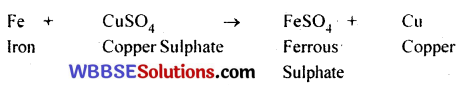Question 17.
What is double displacement reaction? Give example.
The chemical reaction in which two compounds exchange their radicals is called a double displacement reaction.
For example –
If dilute hydrochloric acid (HCl) is added to a solution of Silver Nitrate, a white insoluble solid (Silver Chloride) is formed.Question 18.
What information we can get from symbol CO2 ?